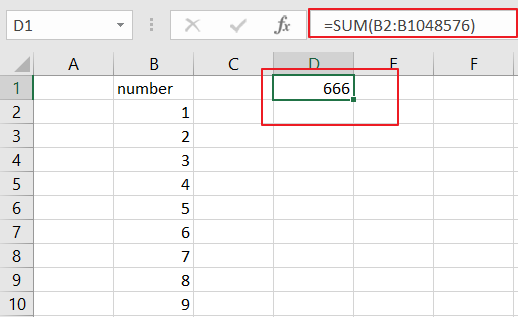# How To Sum Entire Column Except for Certain Rows(Header or First Row) in Excel

This post will guide you how to sum entire column except header or first row value in your worksheet in Excel. How do I sum entire column in excel except for certain rows with a formula in Excel.

Assuming that you want the sum of an entire column but the first row or the header row, and no matter how many entries a column is.

For Excel 2013, and you can use the following formula based on the SUM function to sum entire column except the first cell, type:

=SUM(B2:B1048576)

Or

=SUM(B:B)-SUM(B1:B1)

Or

=SUM(B: B)- B1

Note: if you want to sum an entire column without supplying an upper or lower bound, and you can use an formula based on the SUM function, type:

=SUM(B:B)

And if you want to sum all of row 1, and you can use the following formula:

=SUM(1:1)

B:B will select the entire column B and 1:1 will select the entire row 1.### Related Functions

• Excel SUM function
The Excel SUM function will adds all numbers in a range of cells and returns the sum of these values. You can add individual values, cell references or ranges in excel.The syntax of the SUM function is as below:= SUM(number1,[number2],…)…

Related Posts

Calculate Cumulative Totals with Excel SUM Function

Today, through a simple example, we will show you how to use one of the most common-used Mathematical functions in excel, the SUM function, to add up the sum. In our daily life, we keep an account of what we ...

Check Dates in chronological order

Assume you have a date list that has different date formats, as seen in the accompanying picture. In this instance, Excel's Sort function will fail to sort them appropriately. However, you may convert all various date formats to a particular ...

How to Use 3D SUM Multiple Worksheets

To sum a range of numbers is straightforward for most Excel users, but do you know how to establish a 3D reference to total the same range of numerous sheets. In this post, I will present the steps for this ...

If Cell Begins with One of Three Supplied Characters

If you want to test values to see if they begin with some given specific characters like “x”, ”y”, or “z”, you can create a formula with COUNTIF and SUM functions to return results. EXAMPLE You can see “TRUE” or ...

Average per Week by Formula in Excel

We usually apply AVERAGE function or relevant functions to return average directly in Excel worksheet. But in some situations, only applying average relevant functions cannot figure out our problem. Sometimes we can create a formula with functions and mathematical operation ...

How to Sum if Contains an Asterisk

To add numbers together we need to apply SUM function. And if we want to add numbers based on some conditions, we can add criteria with the help of SUMIFS function, SUMIFS can filter data with multiple criteria effectively. If ...

How to Sum in Vertical Range

If we want to add numbers based on some conditions in Excel worksheet, we can add criteria with the help of SUMIFS function, SUMIFS can filter data with multiple criteria effectively. In this article, we will introduce you the method ...

How to Sum in Horizontal Range

To add numbers together we need to apply SUM function. But if we want to add numbers based on some conditions, we can add criteria with the help of SUMIFS function, SUMIFS can filter data with multiple criteria effectively. In ...

How to Sum with Criteria and Or Logic in Excel

To add numbers together we need to apply SUM function. And if we want to add numbers based on some conditions, we can add criteria with the help of SUMIFS function, SUMIFS can filter data with multiple criteria effectively. If ...

How To Sum the Largest N Values in Excel

Sometimes we may want to sum the largest N numbers or top N numbers in a range. In this article, we will show you the method of “SUM the Largest N Numbers” by a simple formula which consist of SUMPRODUCT ...

Sidebar